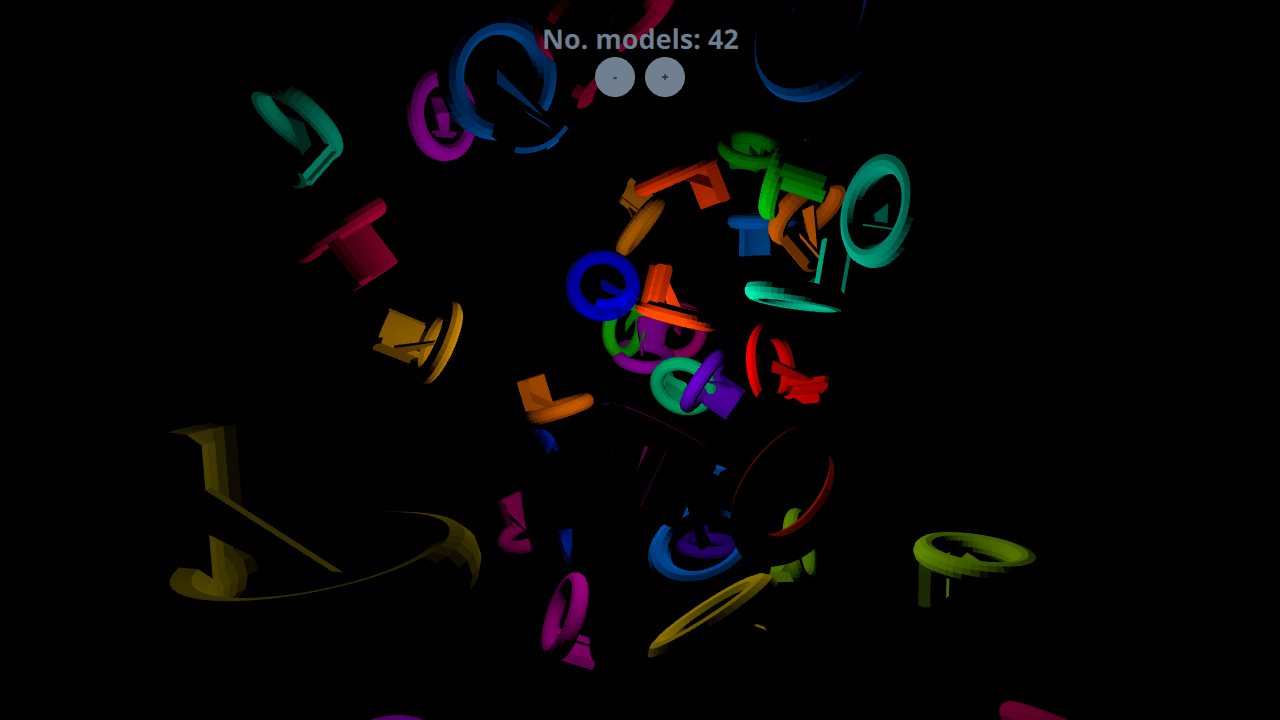# Qt Quick 3D - Dynamic Model Creation Example

Demonstrates dynamic model creation.

This example demonstrates creating models dynamically in an application. 10 models are created dynamically at the start of the application, and more can be added or removed using the + and - buttons.#### Setting Up

##### Spawner Node

We are going to need a Node to hold the dynamically created models.

```Node {
id: shapeSpawner
property real range: 300
property var instances: []
property int count
...```
##### Startup

We're creating 10 models at `Component.onCompleted` so the example shows something at startup.

```Component.onCompleted: {
for (var i = 0; i < 10; ++i)
}```

#### Dynamic Models

In order to add a new item to the scene, we first use the Qt.createComponent function to create a Component for our model. Then we use the component's createObject function to instantiate the item, passing in the position and scale as parameters.

```function addShape()
{
var xPos = (2 * Math.random() * range) - range;
var yPos = (2 * Math.random() * range) - range;
var zPos = (2 * Math.random() * range) - range;
var shapeComponent = Qt.createComponent("WeirdShape.qml");
let instance = shapeComponent.createObject(shapeSpawner,
{ "x": xPos, "y": yPos, "z": zPos, "scale": Qt.vector3d(0.25, 0.25, 0.25)});
instances.push(instance);
count = instances.length
}```
##### Removing Models

Dynamically created models are removed simply by popping and destroying them from the instance stack.

```function removeShape()
{
if (instances.length > 0) {
let instance = instances.pop();
instance.destroy();
count = instances.length
}
}```

Files: